Courses

# Test: Discrete-Circuit MOS Amplifiers

## 10 Questions MCQ Test Electronic Devices | Test: Discrete-Circuit MOS Amplifiers

Description
This mock test of Test: Discrete-Circuit MOS Amplifiers for Electrical Engineering (EE) helps you for every Electrical Engineering (EE) entrance exam. This contains 10 Multiple Choice Questions for Electrical Engineering (EE) Test: Discrete-Circuit MOS Amplifiers (mcq) to study with solutions a complete question bank. The solved questions answers in this Test: Discrete-Circuit MOS Amplifiers quiz give you a good mix of easy questions and tough questions. Electrical Engineering (EE) students definitely take this Test: Discrete-Circuit MOS Amplifiers exercise for a better result in the exam. You can find other Test: Discrete-Circuit MOS Amplifiers extra questions, long questions & short questions for Electrical Engineering (EE) on EduRev as well by searching above.
QUESTION: 1

Solution:
QUESTION: 2

### (Q.2-Q.6) The MOSFEt circuit below has Vt = 1V, knW/L = 0.8 mA/V2 and VA = 40V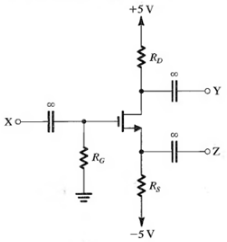Find the value of RG so that iD = 0.1 mA, the largest possible value of RD is used while the maximum signal swing at the drain is of 1V and the input resistance at the gate is 10 MΩ.

Solution: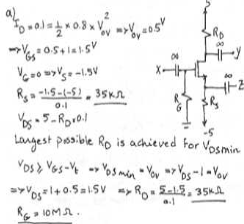QUESTION: 3

### Find the value of gm at the bias point

Solution:
QUESTION: 4

If terminal Z is grounded, X is connected to a signal source having a resistance of 1 MΩ and terminal Y is connected to a load resistance of 40 kΩ, find the voltage gain from the signal source to the load.

Solution:
QUESTION: 5

If terminal Y is grounded find the voltage gain from X to Z with Z open-circuit.

Solution:
QUESTION: 6

If terminal X is grounded and terminal Z is connected to a current source delivering a current of 10 µA and having a resistance of 100 kΩ, find the voltage signal that can be measured at Y neglecting the effect of V0 .

Solution:
QUESTION: 7

(Q.7-Q.8) The NMOS transistor in source follower circuit shown has gm = 5mA/V and a large r0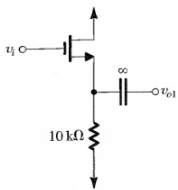Q. Find the output resistance.

Solution:
QUESTION: 8

Find the open-Circuit voltage gain.

Solution:
QUESTION: 9

The NMOS transistor in the common gate amplifier as shown in the circuit below has gm = 5 mA/V. Find the input resistance and the voltage gain.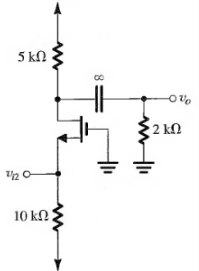Solution:
QUESTION: 10

If the output of the source follower in (I) is connected to the input of the common gate amplifier of (II). Determine the overall voltage gain (V0 /Vi ).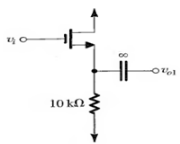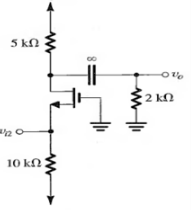Solution: